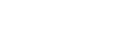# Get the value of the last non blank cell in a column in Microsoft Excel

In this article, we will learn how to get the value to the last non-blank cell in a column.

To get the last non-blank value we will use COUNTA  function along with OFFSET function in Microsoft Excel.

COUNTA: To return the count of the number of cells that contain values, you can use the function COUNTA.

Syntax of “COUNTA” function: =COUNTA (value1, value2, value3…….)

Example: In range A1:A5, cells A2, A3 and A5 contain the values, and cells A1 and A4 are blank. Select the cell A6 and write the formula “COUNTA” to count the nonblank cells.

=COUNTA(A1:A5)the function will return 3One of the rows and columns in a range that is a specified number of the cell or range of cells has a reference returned references. A single cell or range of cells may have to be returned to the number of rows and number of columns can be specified.

Syntax of OFFSET function:    = OFFSET (reference, rows, cols, height, width)

Reference: - This is the cell or range from which you want to offset.

Rows and Columns to move: - How many rows you want to move the starting point and both of these can be positive, negative or zero.

Height and Width: - This is the size of the range you want to return.

Let’s take an example to understand how the Offset function works in Excel:-

We have data in range A1:D10 in which column A contains Product Code, Column B contains Quantity, column C contains per product cost and column D contains Total cost. Now, we want to return the value of cell C5 in cell E2.• Select the cell E2 and write the formula.
• =OFFSET(A1,4,2,1,1)
• Press Enter on your keyboard.
• The function will return the value of cell C5.In this article, ‘COUNTA” function will help us to count the non-blank cells and offset formula will be used to pick the value as per the cell reference.

Let’s take an example to understand how we can get the value of the last non blank cell in a column.

We have month list in column A. Now, we want to find out that what value last non blank cell contains.• Write the formula in cell B2.
• =OFFSET(A1,COUNTA(A:A)-1,0)
• Press Enter on your keyboard.
• The function will return the value of last non blank cell.This is the way by which we can get the value of the last non-blank cell in a column in Microsoft Excel.We would love to hear from you, do let us know how we can improve, complement or innovate our work and make it better for you. Write us at info@exceltip.com

1.Appreciate if you could help me with a formula to achieve the intent shown below.

Original array Intended array Intended logic
2 2 A2"" so A2
2 A3="" update previous non-blank value i.e. A2
2 A4="" update previous non-blank value i.e. A2
4 4 A5"" so A5
4 A6="" update previous non-blank value i.e. A5
6 6 A7"" so A7
6 A8="" update previous non-blank value i.e. A5
6 A9="" update previous non-blank value i.e. A5
6 A10="" update previous non-blank value i.e. A5
8 8 A11"" so A11

2.Works perfectly for my need. Thanks!

3.Alas, this formula does not work if any of the cells in A1:A12 are empty, which is what I'm dealing with.

4.Very good. Thx!

5.Is there a way to get the value of the last cell with text in a column with blank cells in the column of data

Terms and Conditions of use

The applications/code on this site are distributed as is and without warranties or liability. In no event shall the owner of the copyrights, or the authors of the applications/code be liable for any loss of profit, any problems or any damage resulting from the use or evaluation of the applications/code.